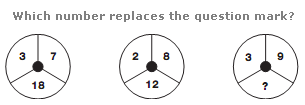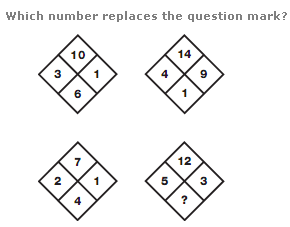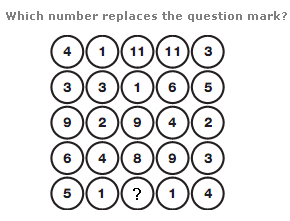# Puzzles - Number puzzles

Exercise : Number puzzles
31.:
22
Explanation
:
In each circle, the lower number equals the product of the top two numbers, subtracting 3 for the left hand circle, 4 for the middle, and 5 for the right hand circle.

32.:
4
Explanation
:
In each diamond, add the left and right hand numbers together, and subtract this sum from the top number to give the value at the bottom.

33.